Search
Document Center

# Deep Learning Network Visualization Development

Last Updated: Jul 21, 2019
``In the deep learning field, graphs are always better than mathematical formulas.``This topic describes how to use the fast neural network function of DSW to visualize deep learning networks.``

Neural networks were first introduced to the biological field to form a network of nerves in the brain. Then mathematical formulas were used to simulate the procedure of how the brain analyzing objects. Later, the deep learning framework was introduced. Scientists write code to build deep learning networks. Complex deep learning networks always contain tens or even hundreds of rows of code. Each network layer also contains many parameters, as shown in the following figure: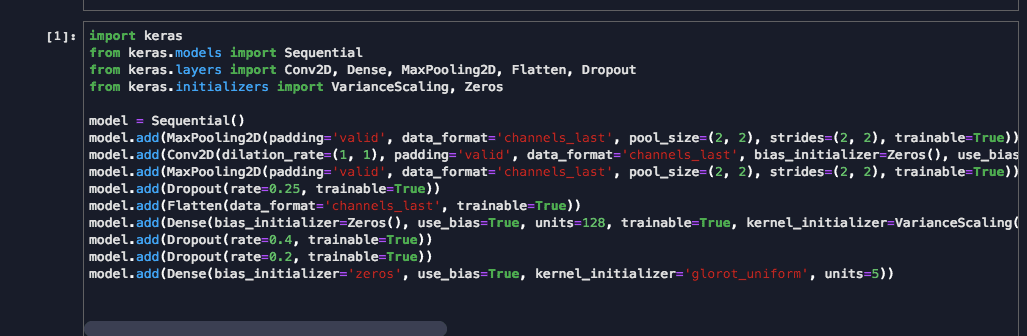As more network layers are added, it is hard to build, modify, and maintain the deep learning networks by writing code. The fast neural network function is used to convert the deep learning network code to a network framework graph for visual editing. It makes the models easier to read and maintain, as shown in the following figure: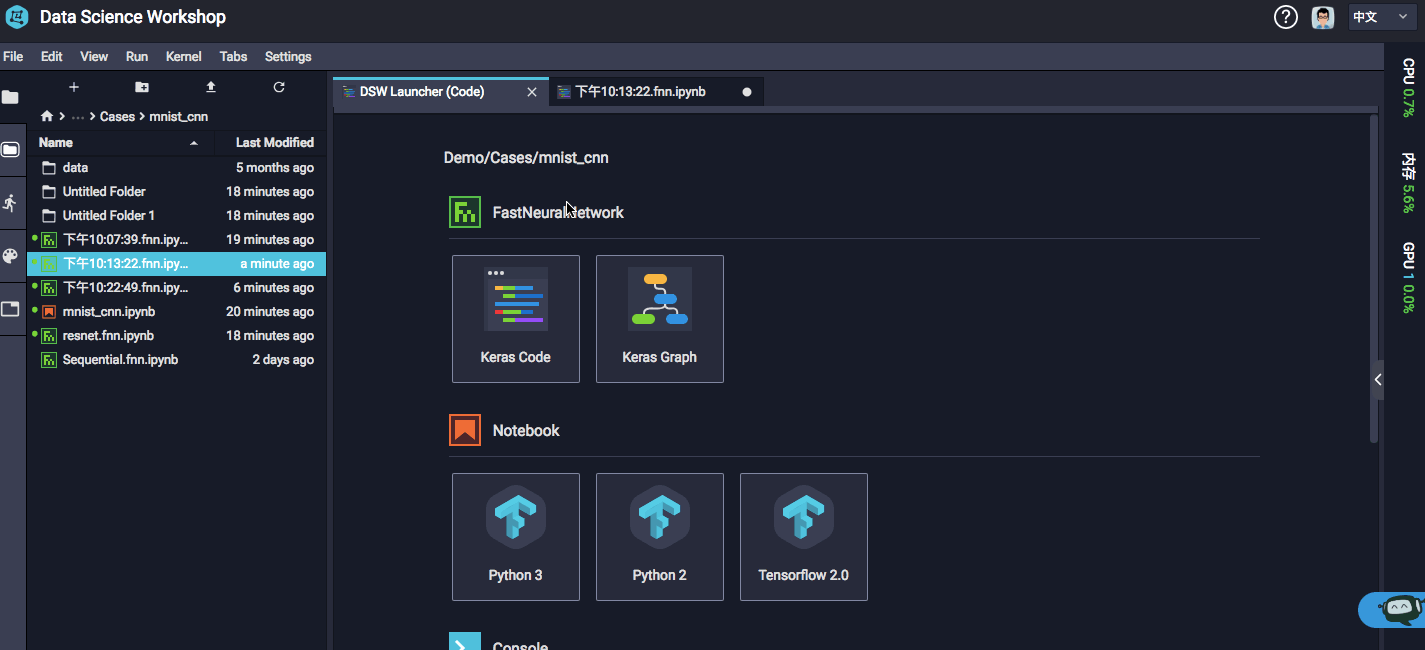The following describes how to use the fast neural network function.

# Features

## 1. Create a graph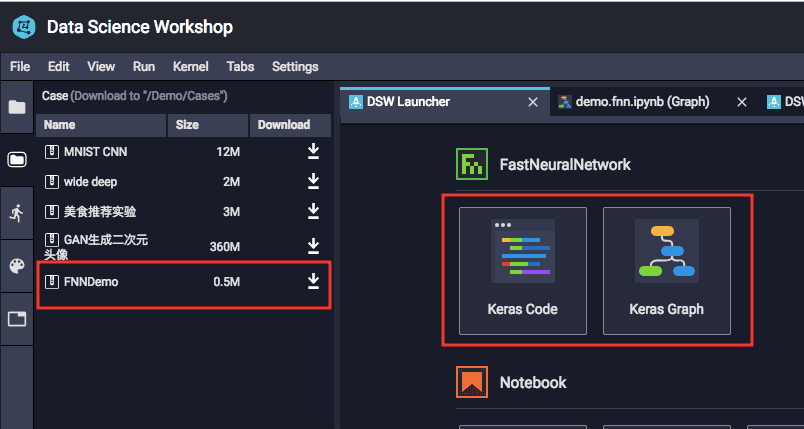Log on to DSW. Currently, only the Keras Code and Keras Graph kernels support the fast neural network function.

• Keras Code: Write deep learning network code and then convert the code to a graph.
• Keras Graph: Build a deep learning network on the canvas and then convert the graph to code.

To use the demo FNNDemo provided by Keras, click Demos in the left-side navigation pane and then select the demo.

## 2. Magic commands

Open Keras Code to go to the interactive development page, and write code to build a deep learning network. The sample code is as follows:

``import keras``from keras.models import Model``from keras.models import Sequential``from keras.layers import Conv2D, Dense, MaxPooling2D, Flatten, Dropout``from keras.initializers import VarianceScaling, Zeros``model = Sequential()``model.add(MaxPooling2D(padding='valid', data_format='channels_last', pool_size=(2, 2), strides=(2, 2), trainable=True))``model.add(Conv2D(dilation_rate=(1, 1), padding='valid', data_format='channels_last', bias_initializer=Zeros(), use_bias=True, filters=64, strides=(1, 1), trainable=True, kernel_initializer=VarianceScaling(mode='fan_avg', seed=None, scale=1.0, distribution='uniform'), activation='relu', kernel_size=(3, 3)))``model.add(MaxPooling2D(padding='valid', data_format='channels_last', pool_size=(2, 2), strides=(2, 2), trainable=True))``model.add(Dropout(rate=0.25, trainable=True))``model.add(Flatten(data_format='channels_last', trainable=True))``model.add(Dense(bias_initializer=Zeros(), use_bias=True, units=128, trainable=True, kernel_initializer=VarianceScaling(mode='fan_avg', seed=None, scale=1.0, distribution='uniform'), activation='relu'))``model.add(Dropout(rate=0.4, trainable=True))``model.add(Dropout(rate=0.2))``

The sample code builds a sequential model. The object is model. You can enter the following magic command to convert the code to a graph:

``%show_model model``

Click the graph to open the graph editing page: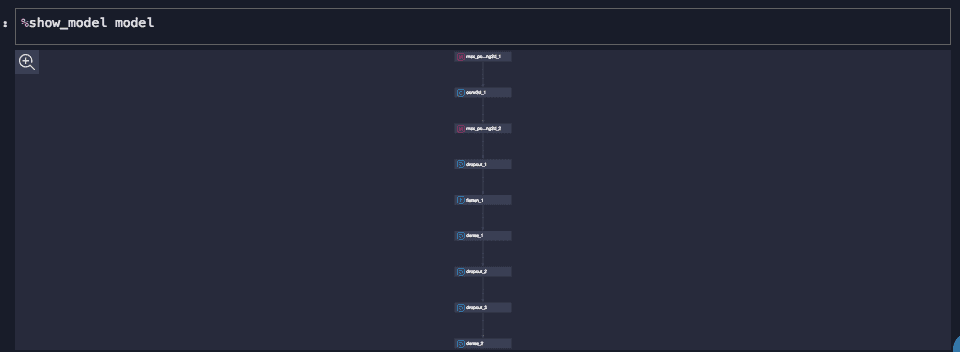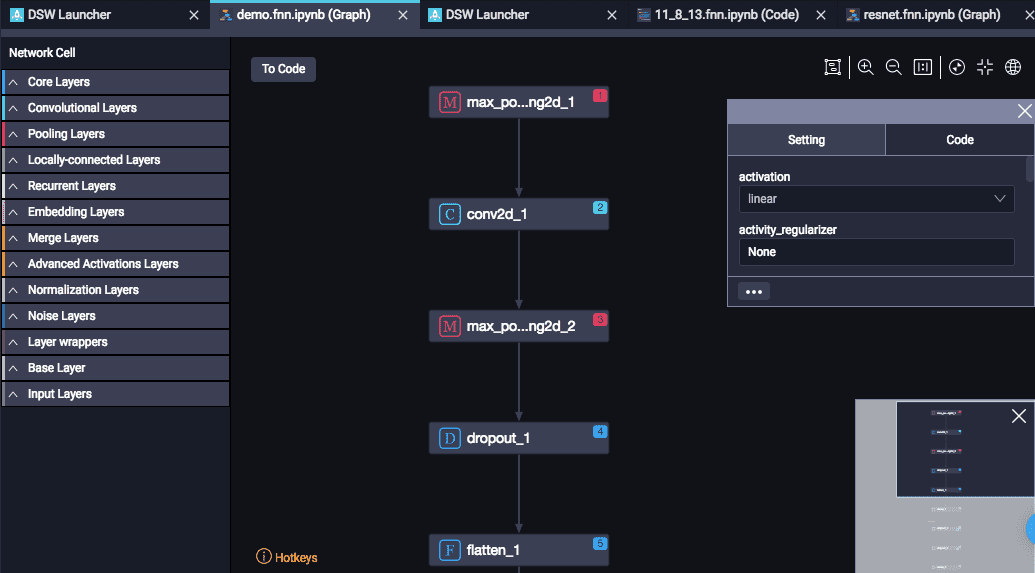## 3. Edit the deep learning network

The Fast Nearest Neighbor (FNN) function allows you to drag, drop, and edit native Keras cells. The graph editing page contains the cells section, canvas section, and parameters section.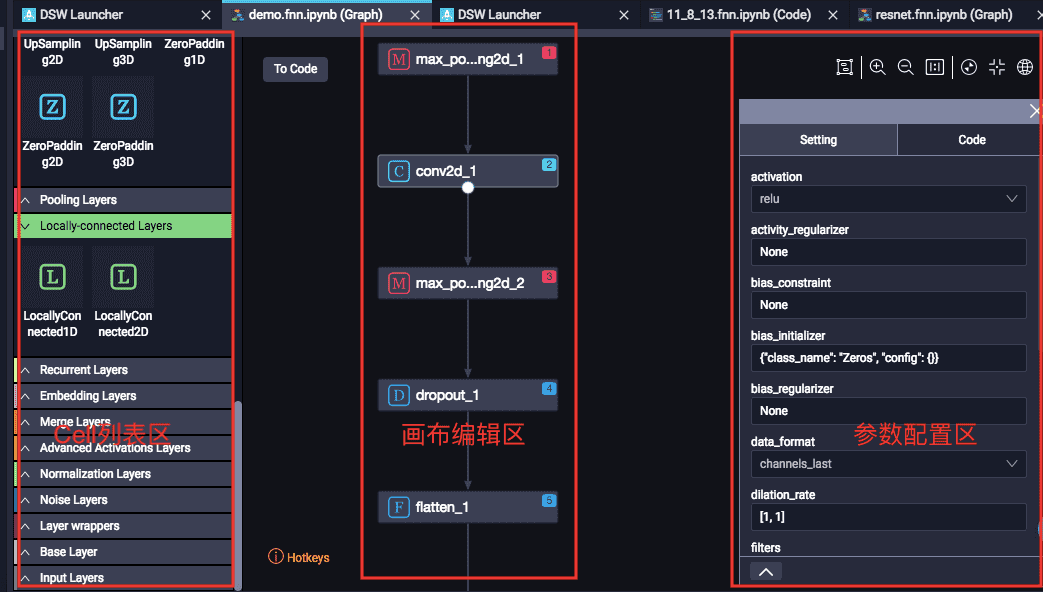Cells with the same function are automatically grouped.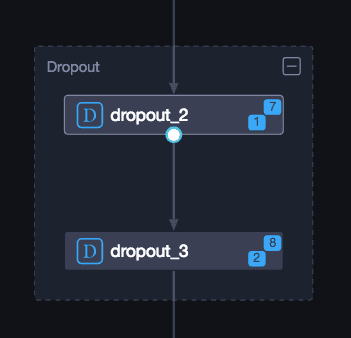The cells in the canvas are automatically mapped to the code: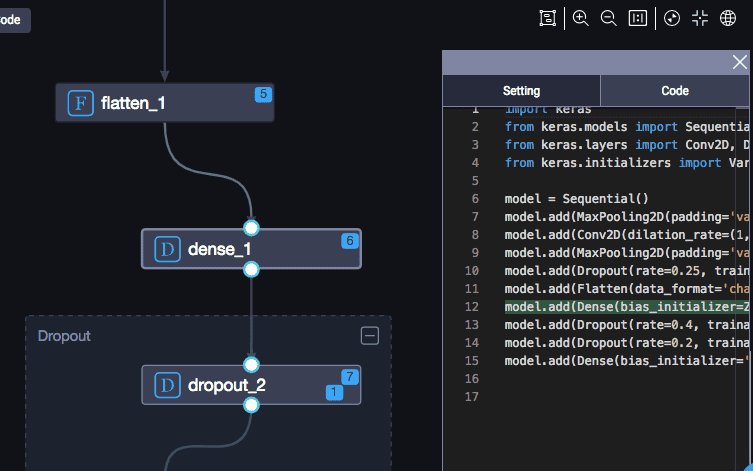## 4. Save the code

Click To Code in the upper-left corner of the canvas, the system then shows the original code and the modified code: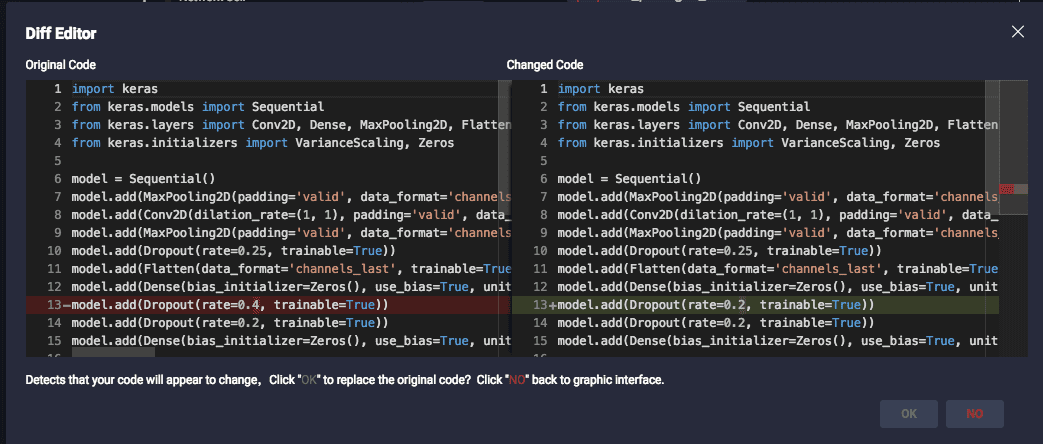Click OK to apply the changes to the code file.